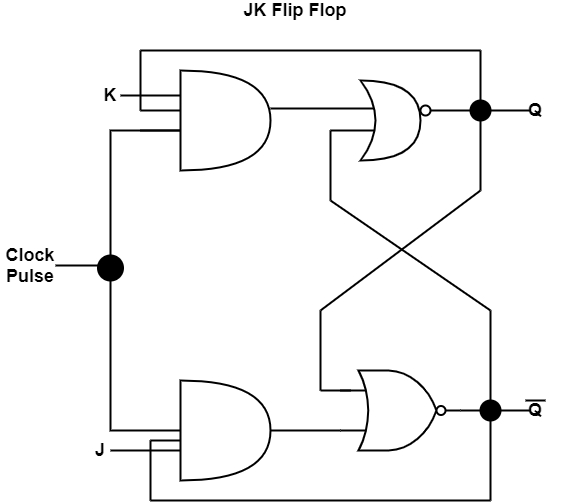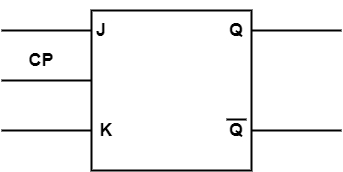# What is J-K Flip Flop?

J-K flip-flop can be treated as an alteration of the S-R flip-flop. J represents SET, and 'K' represents CLEAR. In the JK flip-flop, the ‘S’ input is known as the ‘J’ input, and the ‘R’ input is known as the ‘K’ input. The output of the JK flip-flop does not modify if both ‘J’ and ‘K’ are ‘0’. If both the inputs are ‘1’, then the output dial to its free.

The figure shows the circuit diagram of a JK flip-flop.The truth table of the JK flip-flop is displayed in the table.

SRQN-1
00QN
010
101
11$\overline{Q_{N}}$

The logic symbol for the JK flip-flop is demonstrated in the diagram.